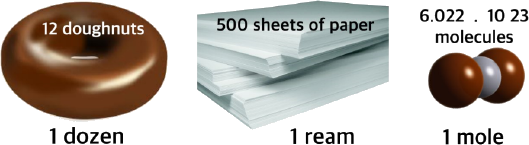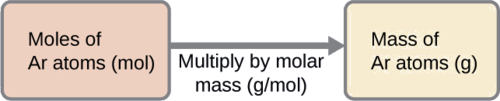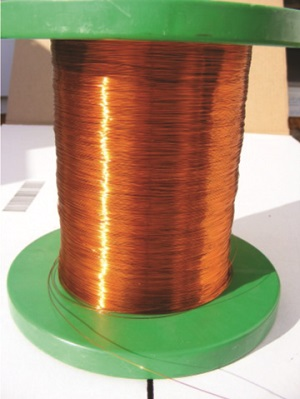# 2.8: Atoms and the Mole - How Many Particles?

Dalton’s theory that each chemical compound has a particular combination of atoms and that the ratios of the numbers of atoms of the elements present are usually small whole numbers. It also describes the law of multiple proportions, which states that the ratios of the masses of elements that form a series of compounds are small whole numbers. The problem for Dalton and other early chemists was to discover the quantitative relationship between the number of atoms in a chemical substance and its mass. Because the masses of individual atoms are so minuscule (on the order of 10−23 g/atom), chemists do not measure the mass of individual atoms or molecules. In the laboratory, for example, the masses of compounds and elements used by chemists typically range from milligrams to grams, while in industry, chemicals are bought and sold in kilograms and tons. To analyze the transformations that occur between individual atoms or molecules in a chemical reaction, it is therefore essential for chemists to know how many atoms or molecules are contained in a measurable quantity in the laboratory—a given mass of sample.

## The Mole: A Chemistry "Dozen"

Because atoms and molecules are extremely small, there are a great many of them in any macroscopic sample. For example a 1 cm3 of mercury would contain $$4.080 \times 10^{22}$$ mercury atoms. The very large numbers involved in counting microscopic particles are inconvenient to think about or to write down. Chemists have chosen to count atoms and molecules using a unit called the mole (mol), from the Latin moles, meaning “pile” or “heap.” One mole is 6.022 x 1023 of the microscopic particles which make up the substance in question. Thus 6.022 x 1023 Br atoms is referred to as 1 mol Br.

The mole is an amount unit similar to familiar units like pair, dozen, gross, etc. It provides a specific measure of the number of atoms or molecules in a bulk sample of matter. The mole provides a link between an easily measured macroscopic property, bulk mass, and an extremely important fundamental property, number of atoms, molecules, and so forth.

The number of entities composing a mole has been experimentally determined to be $$6.02214179 \times 10^{23}$$. This is a fundamental constant named Avogadro’s number ($$N_A$$) or the Avogadro constant in honor of Italian scientist Amedeo Avogadro. This constant is properly reported with an explicit unit of “per mole,” a conveniently rounded version being $$6.022 \times 10^{23}/\ce{mol}$$.

Using Units of Specific Number of Items is Common

Many familiar items are sold in numerical quantities with distinct names. For example, cans of soda come in a six-pack, eggs are sold by the dozen (12), and pencils often come in a gross (12 dozen, or 144). Sheets of printer paper are packaged in reams of 500, a seemingly large number. Atoms are so small, however, that even 500 atoms are too small to see or measure by most common techniques. Any readily measurable mass of an element or compound contains an extraordinarily large number of atoms, molecules, or ions, so an extremely large numerical unit is needed to count them.Figure $$\PageIndex{1}$$: Examples of using a specific number as a unit of measurement.

There is a difference in degree, however, because the mole of anything (6.022 x 1023) is so large. A stack of paper containing a mole of sheets would extend more than a million times the distance from the earth to the sun, and 6.022 x 1023 grains of sand would cover all the land in the world to a depth of nearly 2 ft. Obviously there are a great many particles in a mole of anything.

A mole is formally defined as the amount of substance containing the same number of discrete entities (such as atoms, molecules, and ions) as the number of atoms in a sample of pure 12C weighing exactly 12 g. Consistent with this definition, 1 mole of any element contains the same number of atoms as 1 mole of any other element. The masses of 1 mole of different elements, however, are different, since the masses of the individual atoms are drastically different. The molar mass of an element (or compound as we will demonstrate) is the mass in grams of 1 mole of that substance, a property expressed in units of grams per mole (g/mol) (Figure $$\PageIndex{2}$$).Figure $$\PageIndex{2}$$: Each sample contains $$6.022 \times 10^{23}$$ atoms —1.00 mol of atoms. From left to right (top row): 65.4 g zinc, 12.0 g carbon, 24.3 g magnesium, and 63.5 g copper. From left to right (bottom row): 32.1 g sulfur, 28.1 g silicon, 207 g lead, and 118.7 g tin. (credit: modification of work by Mark Ott).

Because the definitions of both the mole and the atomic mass unit are based on the same reference substance, 12C, the molar mass of any substance is numerically equivalent to its atomic mass in amu. Per the amu definition, a single 12C atom weighs 12 amu (its atomic mass is 12 amu). According to the definition of the mole, 12 g of 12C contains 1 mole of 12C atoms (its molar mass is 12 g/mol). This relationship holds for all elements, since their atomic masses are measured relative to that of the amu-reference substance, 12C (Table $$\PageIndex{1}$$).

Table $$\PageIndex{1}$$: Mass of one mole of elements
Element Average Atomic Mass (amu) Atomic Mass (g/mol) Atoms/Mole
C 12.01 12.01 $$6.022 \times 10^{23}$$
H 1.008 1.008 $$6.022 \times 10^{23}$$
O 16.00 16.00 $$6.022 \times 10^{23}$$
Na 22.99 22.99 $$6.022 \times 10^{23}$$
Cl 33.45 35.45 $$6.022 \times 10^{23}$$

Overview of Origin and Properties of the Mole

The word "mole" suggests a small, furry burrowing animal to many. But in this lesson, we look at the concept of the mole in chemistry. Learn the incredible magnitude of the mole--and how something so big can help us calculate the tiniest particles in the world.

## Converting between Number of Moles and Number of Atoms

Although chemists usually work with moles as units, occasionally it is helpful to refer to the actual number of atoms or molecules involved. When this is done, the symbol $$N$$ is used for the number of species and $$n$$ is used for the number of moles. For example, in referring to 1 mol of helium atoms, we could write

$n_{\ce{He}} = 1 \text{mol} \nonumber$

and

$N_{\ce{He}} = 6.022\times 10^{23} \nonumber$

Obtaining $$N$$ requires the use of a conversion factor to obtain, which is just $$N_A$$. This which is defined by the equation

$N_{\text{A}} = \dfrac{N}{n} \label{eq1}$

Since for any substance there are 6.022 × 1023 particles per mole, Equation \ref{eq1} can be expanded:

$\textit{N}_\text{A}=\dfrac{6.022\cdot10^{23}} {1\text{ mol}}=6.022\times 10^{23}\text{ mol}^{\text{–1}} \label{eq2}$

Equation \ref{eq2} is the most important conversion factor in general chemistry. Figure $$\PageIndex{3}$$ is a flowchart for converting between the number of moles and the number of atoms. The use of these conversions is illustrated in Example $$\PageIndex{1}$$ and Exercise $$\PageIndex{1}$$.Figure $$\PageIndex{3}$$: Forward and Reverse Flowcharts for Converting between the Number of Moles and the Number of Atoms (CC BY-NC-SA 3.0; anonymous)

Example $$\PageIndex{1}$$: Copper Atoms

How many atoms are present in 2.76 mol of copper atoms?

Solution

The definition of a mole is an equality that can be used to construct a conversion factor.

$2.76\, \cancel{mol\, \ce{Cu}}\times \frac{6.022\times 10^{23}atoms\, \ce{Cu}}{\cancel{mol\, \ce{Cu}}}=1.66\times 10^{24} \text{molecules} \, \ce{Cu} \nonumber$

Exercise $$\PageIndex{1}$$

How many molecules are present in $$4.61 \times 10^{-2}$$ mol of helium atoms?

2.78 × 1022 molecules

## Converting between Mass and Number of Moles

The molar mass of a substance is defined as the mass in grams of 1 mole of that substance. One mole of isotopically pure carbon-12 has a mass of 12 g. For an element, the molar mass is the mass of 1 mol of atoms of that element. That is, the molar mass of a substance is the mass (in grams per mole) of 6.022 × 1023 atoms of that substance. In each case, the number of grams in 1 mol is the same as the number of atomic mass units that describe the atomic mass.

The periodic table lists the atomic mass of carbon as 12.011 amu; the average molar mass of carbon—the mass of 6.022 × 1023 carbon atoms—is therefore 12.011 g/mol.

Reminder: Molar Masses (Atomic Masses) are Weighted Averages of Isotopic Masses

As discussed in Section 1.9, The molar mass of naturally-occurring carbon is different from that of carbon-12 isotope because carbon occurs as a mixture of carbon-12, carbon-13, and carbon-14. One mole of carbon still has 6.022 × 1023 carbon atoms, but 98.89% of those atoms are carbon-12, 1.11% are carbon-13, and a trace (about 1 atom in 1012) are carbon-14. Similarly, the molar mass of uranium is 238.03 g/mol.

The mole is the basis of quantitative chemistry and provides chemists with a way to convert easily between the mass of a substance and the number of individual atoms, molecules, or formula units of that substance. Conversely, it enables chemists to calculate the mass of a substance needed to obtain a desired number of atoms. For example, to convert moles of a substance to mass, the following relationship is used:

$(\text{moles}) \times (\text{molar mass}) \rightarrow \text{mass} \label{3.4.1}$

or, more specifically,

$\cancel{\text{moles}} \times \left ( {\text{grams} \over \cancel{\text{mole}} } \right ) = \text{grams} \nonumber$

Conversely, to convert the mass of a substance to moles:

$\left ( {\text{grams} \over \text{molar mass} } \right ) \rightarrow \text{moles} \label{3.4.2A}$

$\left ( { \text{grams} \over \text{grams/mole}} \right ) = \cancel{\text{grams}} \left ( {\text{mole} \over \cancel{\text{grams}} } \right ) = \text{moles} \label{3.4.2B}$Figure $$\PageIndex{1}$$: Forward and Reverse Flowchart for Converting between Mass; the Number of Moles; and the Number of Atoms. This is expanded version of Figure $$\PageIndex{3}$$(CC BY-NC-SA 3.0; anonymous)

If know the mass and chemical composition of a substance, we can determine the number of moles and calculate number of atoms or molecules in the sample. Likewise, if we know the number of moles of a substance, we can derive the number of atoms or molecules and calculate the substance’s mass.

Example $$\PageIndex{2}$$: Deriving Moles from Grams for an Element

According to nutritional guidelines from the US Department of Agriculture, the estimated average requirement for dietary potassium is 4.7 g. What is the estimated average requirement of potassium in moles?

Solution

The mass of K is provided, and the corresponding amount of K in moles is requested. Referring to the periodic table, the atomic mass of K is 39.10 amu, and so its molar mass is 39.10 g/mol. The given mass of K (4.7 g) is a bit more than one-tenth the molar mass (39.10 g), so a reasonable “ballpark” estimate of the number of moles would be slightly greater than 0.1 mol.

The molar amount of a substance may be calculated by dividing its mass (g) by its molar mass (g/mol):The factor-label method supports this mathematical approach since the unit “g” cancels and the answer has units of “mol:”

$\mathrm{4.7\; \cancel{g} K \left ( \dfrac{mol\; K}{39.10\;\cancel{g}}\right)=0.12\;mol\; K} \nonumber$

The calculated magnitude (0.12 mol K) is consistent with our ballpark expectation, since it is a bit greater than 0.1 mol.

Exercise $$\PageIndex{2}$$: Beryllium

Beryllium is a light metal used to fabricate transparent X-ray windows for medical imaging instruments. How many moles of Be are in a thin-foil window weighing 3.24 g?

0.360 mol

Example $$\PageIndex{3}$$: Deriving Grams from Moles for an Element

A liter of air contains $$9.2 \times 10^{−4}$$ mol argon. What is the mass of Ar in a liter of air?

Solution

The molar amount of Ar is provided and must be used to derive the corresponding mass in grams. Since the amount of Ar is less than 1 mole, the mass will be less than the mass of 1 mole of Ar, approximately 40 g. The molar amount in question is approximately one-one thousandth (~10−3) of a mole, and so the corresponding mass should be roughly one-one thousandth of the molar mass (~0.04 g):In this case, logic dictates (and the factor-label method supports) multiplying the provided amount (mol) by the molar mass (g/mol):

$\mathrm{9.2 \times10^{-4}\; \cancel{mol} \; Ar \left( \dfrac{39.95\;g}{\cancel{mol}\;Ar} \right)=0.037\;g\; Ar} \nonumber$

The result is in agreement with our expectations, around 0.04 g Ar.

Exercise $$\PageIndex{3}$$

What is the mass of 2.561 mol of gold?

504.4 g

Example $$\PageIndex{4}$$: Deriving Number of Atoms from Mass for an Element

Copper is commonly used to fabricate electrical wire (Figure $$\PageIndex{6}$$). How many copper atoms are in 5.00 g of copper wire?Figure $$\PageIndex{6}$$: Copper wire is composed of many, many atoms of Cu. (credit: Emilian Robert Vicol)

Solution

The number of Cu atoms in the wire may be conveniently derived from its mass by a two-step computation: first calculating the molar amount of Cu, and then using Avogadro’s number (NA) to convert this molar amount to number of Cu atoms:Considering that the provided sample mass (5.00 g) is a little less than one-tenth the mass of 1 mole of Cu (~64 g), a reasonable estimate for the number of atoms in the sample would be on the order of one-tenth NA, or approximately 1022 Cu atoms. Carrying out the two-step computation yields:

$\mathrm{5.00\:\cancel{g}\:Cu\left(\dfrac{\cancel{mol}\:Cu}{63.55\:\cancel{g}}\right)\left(\dfrac{6.022\times10^{23}\:atoms}{\cancel{mol}}\right)=4.74\times10^{22}\:atoms\: of\: copper}$

The factor-label method yields the desired cancellation of units, and the computed result is on the order of 1022 as expected.

Exercise $$\PageIndex{4}$$

A prospector panning for gold in a river collects 15.00 g of pure gold. How many Au atoms are in this quantity of gold?

$$4.586 \times 10^{22}\; Au$$ atoms